## Circle-Circle IntersectionLet two Circles of Radii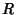andand centered atand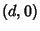intersect in a Lens-shaped region. The equations of the two circles are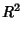(1)(2)

Combining (1) and (2) gives(3)

Multiplying through and rearranging gives(4)

Solving forresults in(5)

The line connecting the cusps of the Lens therefore has half-length given by pluggingback in to obtain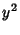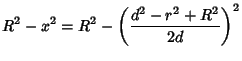(6)

giving a length of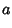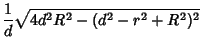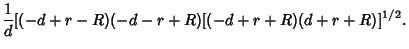(7)

This same formulation applies directly to the Sphere-Sphere Intersection problem.

To find the Area of the asymmetric Lens'' in which the Circles intersect, simply use the formula for the circular Segment of radiusand triangular height(8)

twice, one for each half of the Lens.'' Noting that the heights of the two segment triangles are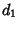(9)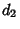(10)

The result is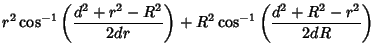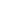(11)

The limiting cases of this expression can be checked to give 0 whenand(12)(13)

when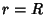, as expected. In order for half the area of two Unit Disks () to overlap, setin the above equation(14)

and solve numerically, yielding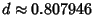.